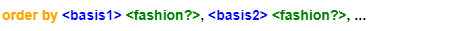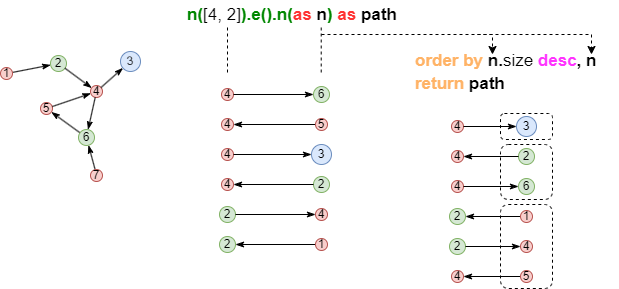# Change Nickname

Current Nickname:

Certifications

Certificate Issued at Valid until Serial No. File
Serial No. Valid until

Not having one? Apply now! >>>

Invoice

ProductName CreateTime ID Price File
ProductName CreateTime ID Price File

# ORDER BY | Sorting

## Format, Parameters

ORDER BY adjusts the order of rows in the data stream.

Syntax:

• Format: order by `<column1>` `<fashion1>`, `<column2>` `<fashion2>`, ...
• Parameters: see table below
• Affected columns: `<column>` and all its homologous columns
Name Category Specification Description
`<column>` NODE，EDGE，PATH，ATTR，ARRAY，TABLE / Sorting basis, multiple basises must be homologous columns and ordering is operated from left to right, from higher levels to lower levels
`<fashion>` string `asc` or `desc`，non-case-sensitive Sorting method, ascending or descending## Analysis

``````find().nodes([4, 2]) as nodes
n(nodes).e().n(as n) as path
return path
``````

In the UQL statement above, `Order By` adjusts the order of the one-step paths resulting from template query: first based on the radii of terminal nodes in descending order, then based on the UUID of terminal nodes that share the same radii in an ascending order.## ATTR (Numeric value)

Example: find 10 cards that have the highest balances, i.e, sorts the card balances in a descinding order

``````find().nodes({@card}) as n
order by n.balance desc
return n{*} limit 10
``````

## ATTR (Time)

Example: find cards of Customer CU001, return cards'info in an ascending time order

``````n({_id == "CU001"}).re({@has}).n({@card} as n)
order by n.open_date
return n{*}
``````

## ATTR (String)

Example: find 10 email addresses, sort them in a descending order of letters from addresses

``````find().nodes({@email}) as n
limit 10
return n{*}
``````

## NODE

Example: find 10 two-step transfer paths, sort paths in a descending order of 2rd nodes' UUIDs

``````n({@card}).e({@transfer}).n({@card} as n)
.e({@transfer}).n({@card}) as p
limit 10
order by n desc
return p{*}
``````

## EDGE

Example: find 10 two-step transfer paths, sort paths in a descending order of the 2rd edges' UUIDs

``````n({@card}).e({@transfer}).n({@card})
.e({@transfer} as e).n({@card}) as p
limit 10
order by e desc
return p{*}
``````

## Group By and Order By

Example: sort all cards in groups by their levels, count the number of cards at each level, return the 3 levels with the most cards and card numbers at these levels

``````find().nodes({@card}) as n
group by n.level as level
return level, count(n) as total limit 3
order by total desc
``````